The code below shows how to visualize different probability density functions (PDF) in R.
It also introduces the following important R concepts, tools and tips:

• You can code and document your code in R (as comments with #) or in Rmd (as regular test). It's often easier to start with R, and then move your codes to Rmd.
• Don't know what function bla-bla() is doing ? - Run ?bla-bla to read the help about it.
• Much better graphs can be using ggplot2 library - just include library(ggplot2) and replace base function plot with qplot!
• It's good practice is to set the seed for random number generation - to make your results reproducible by others.
# File name: prob101_a.R
# Visualizing various probability density functions
# See also: prob101_a.Rmd (the same code inside an automatically generated report)

library(magrittr) # to use ' %>%  '
## Warning: package 'magrittr' was built under R version 4.0.5
# The Normal Distribution

# ?rnorm
# ?Distributions

pnorm(0) # 0.5
##  0.5
pnorm(1) # 0.8413447
##  0.8413447
pnorm(2) # 0.9772499
##  0.9772499
pnorm(3) # 0.9986501
##  0.9986501
# Visualize probability density functions (PDF) and cumulative distribution function (CDF) with different sample points for different distributions

set.seed(111)  # Seed for random number generator. Run ?set.seed to learn about any function you don't know
xx = rnorm(11)
xx = rlnorm(11)
xx = -5:5;
xx = c(-3,-2,-1,-0.5, -0.25, 0, 0.25, 0.5, 1, 2, 3)
xx
##   -3.00 -2.00 -1.00 -0.50 -0.25  0.00  0.25  0.50  1.00  2.00  3.00
# with base graphics
plot(xx, dnorm(xx), main = "Normal PDF = f(x) ")

# with ggplot2 graphics
library(ggplot2)
## Warning: package 'ggplot2' was built under R version 4.0.5
## Registered S3 methods overwritten by 'tibble':
##   method     from
##   format.tbl pillar
##   print.tbl  pillar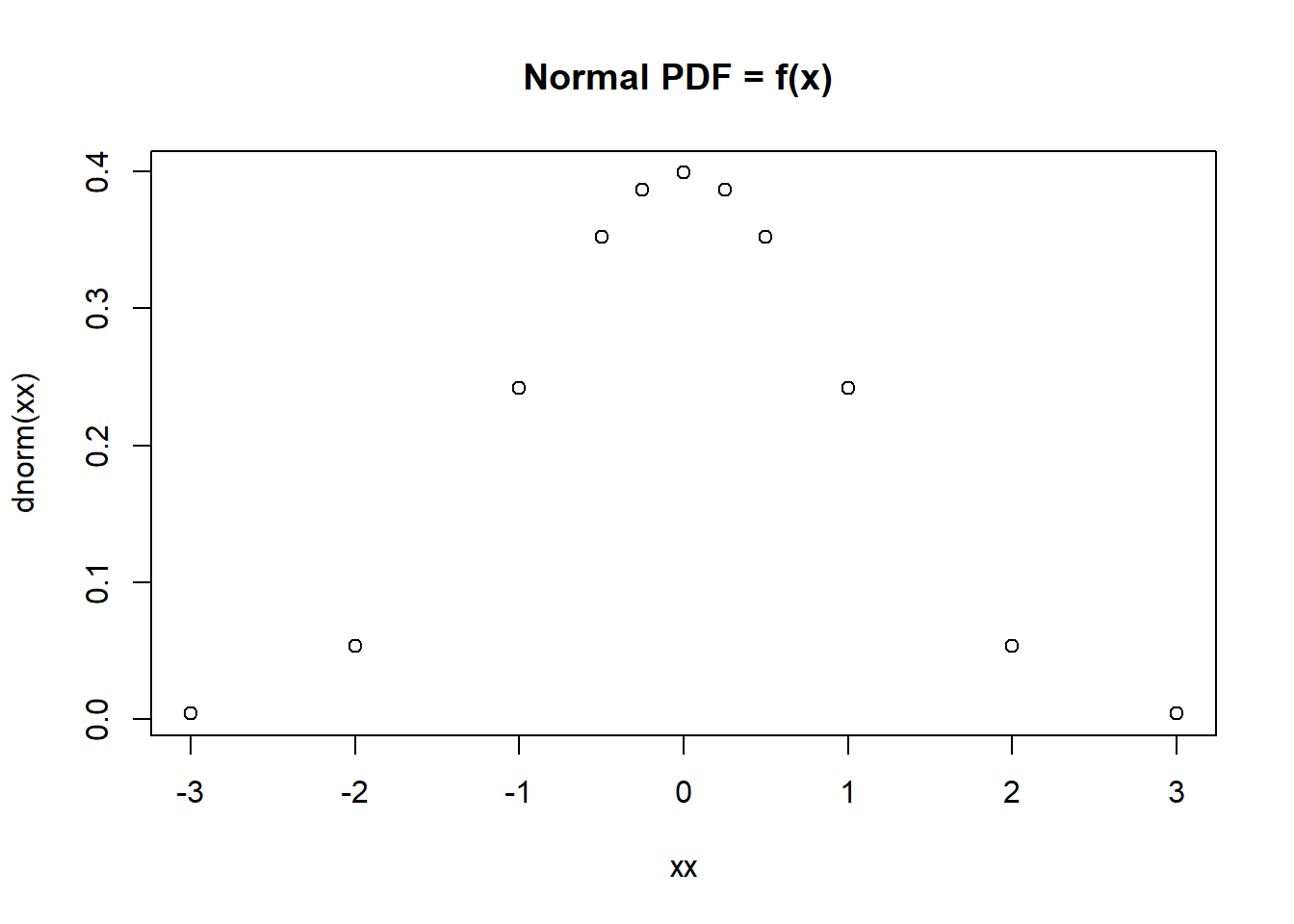qplot(xx, dnorm(xx), geom = c("point", "line"), main = "Normal: PDF = f(x) ")   %>% print()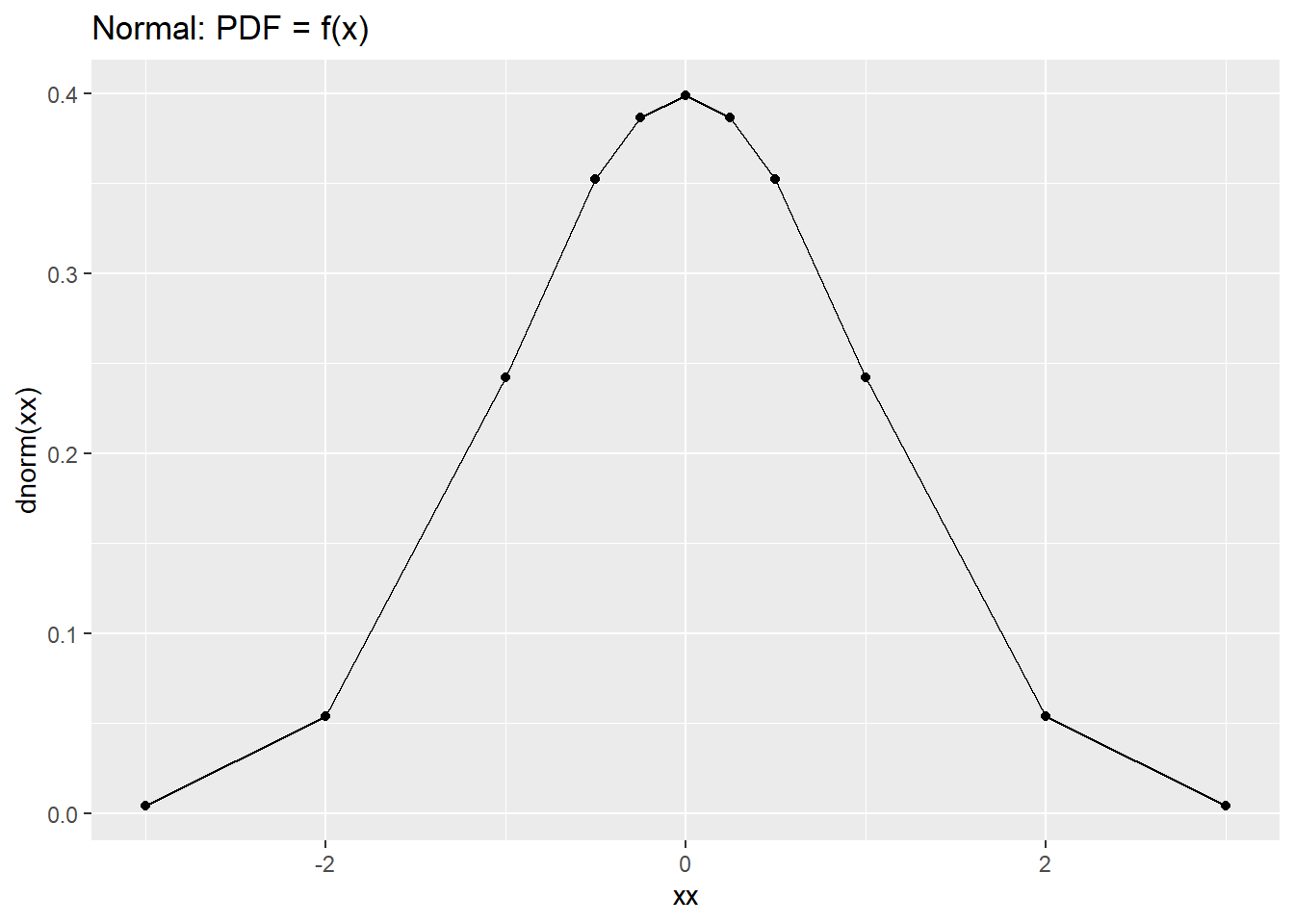qplot(xx, dnorm(xx, log=T), geom = c("point", "line"), main = "Normal: PDF = f(x) ")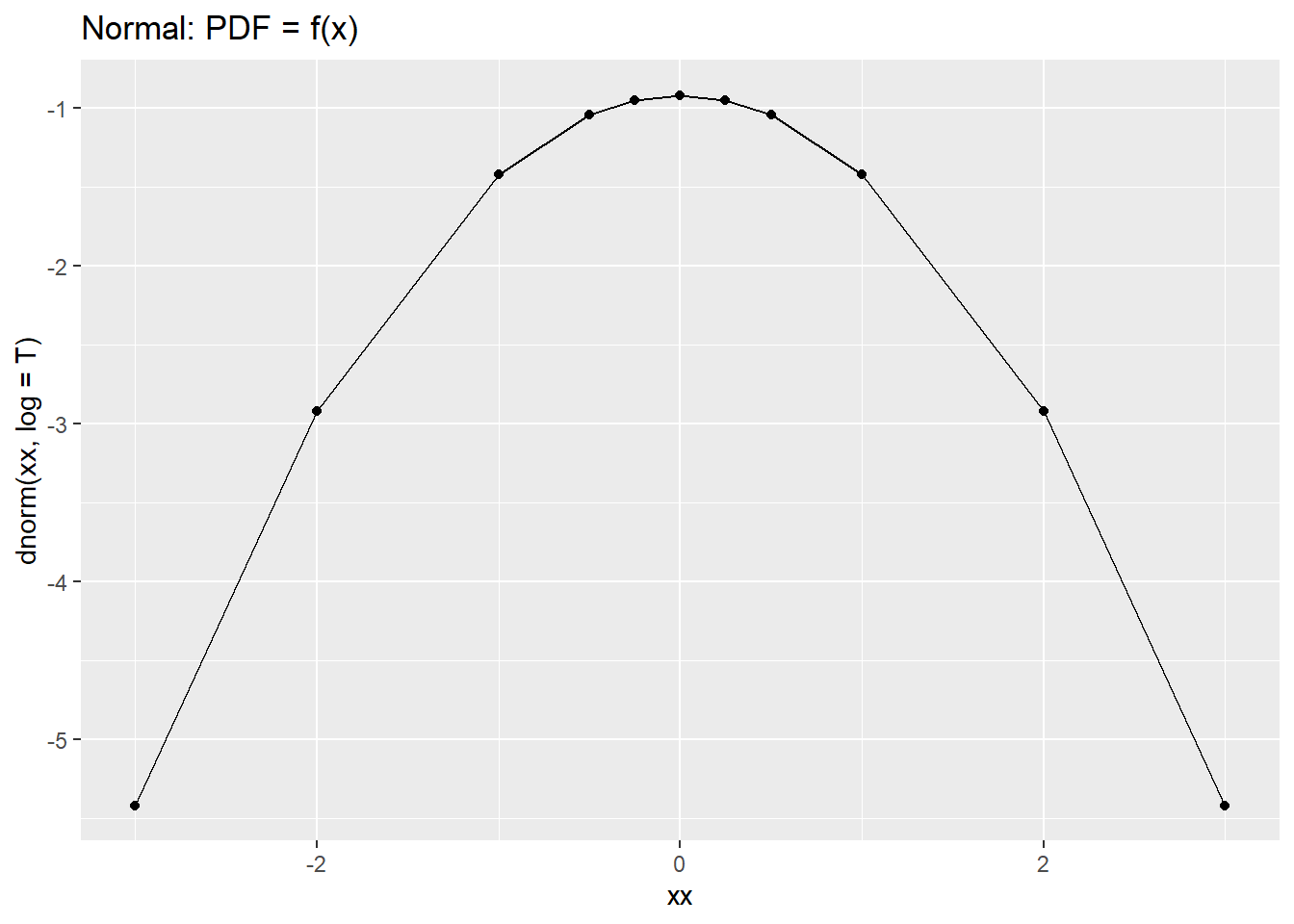qplot(xx, pnorm(xx), geom = c("point", "line"), main = "Normal: CDF = f(x) ")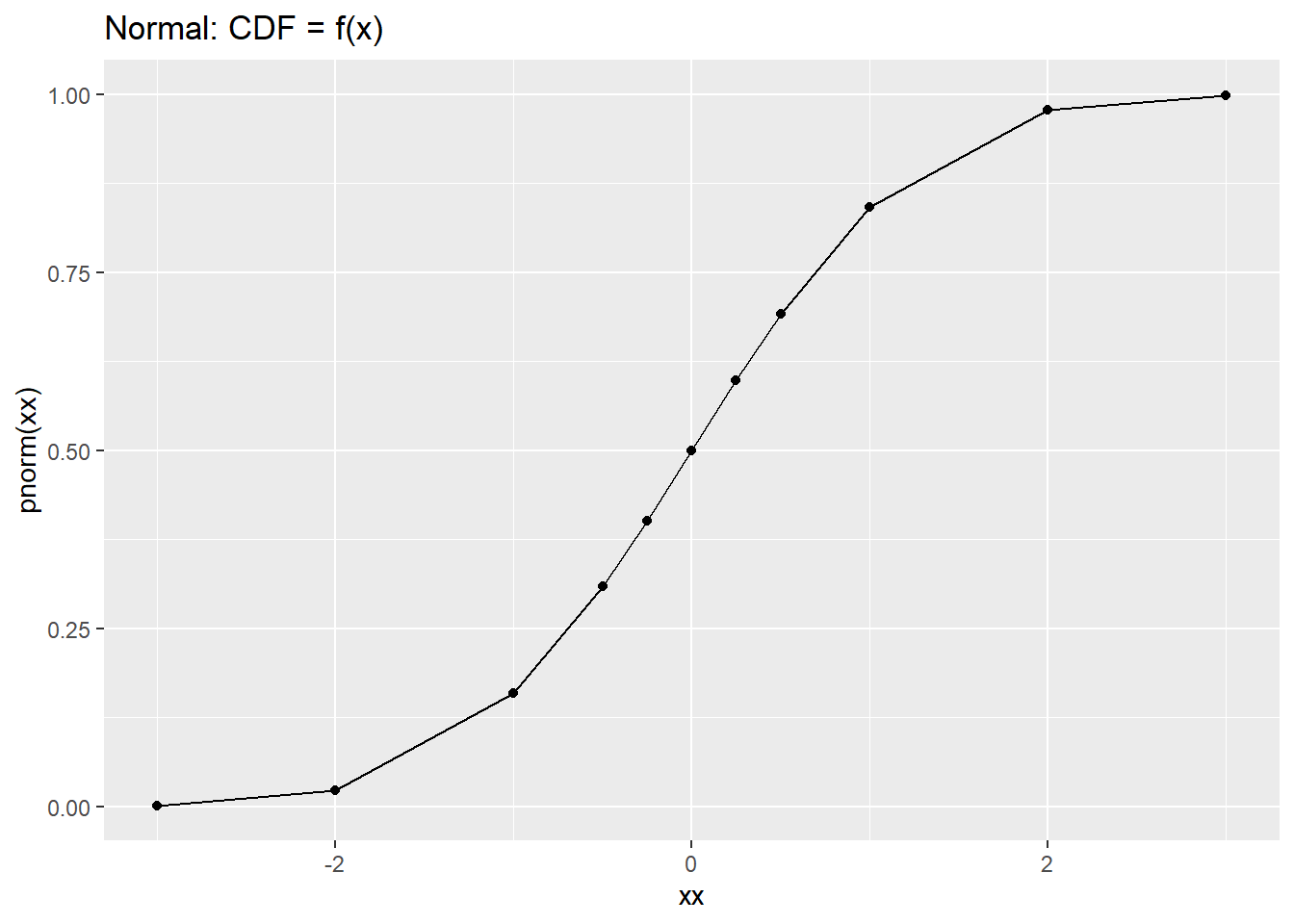qplot(xx, pnorm(xx, log.p=T), geom = c("point", "line"), main = "Normal: CDF = f(x) ")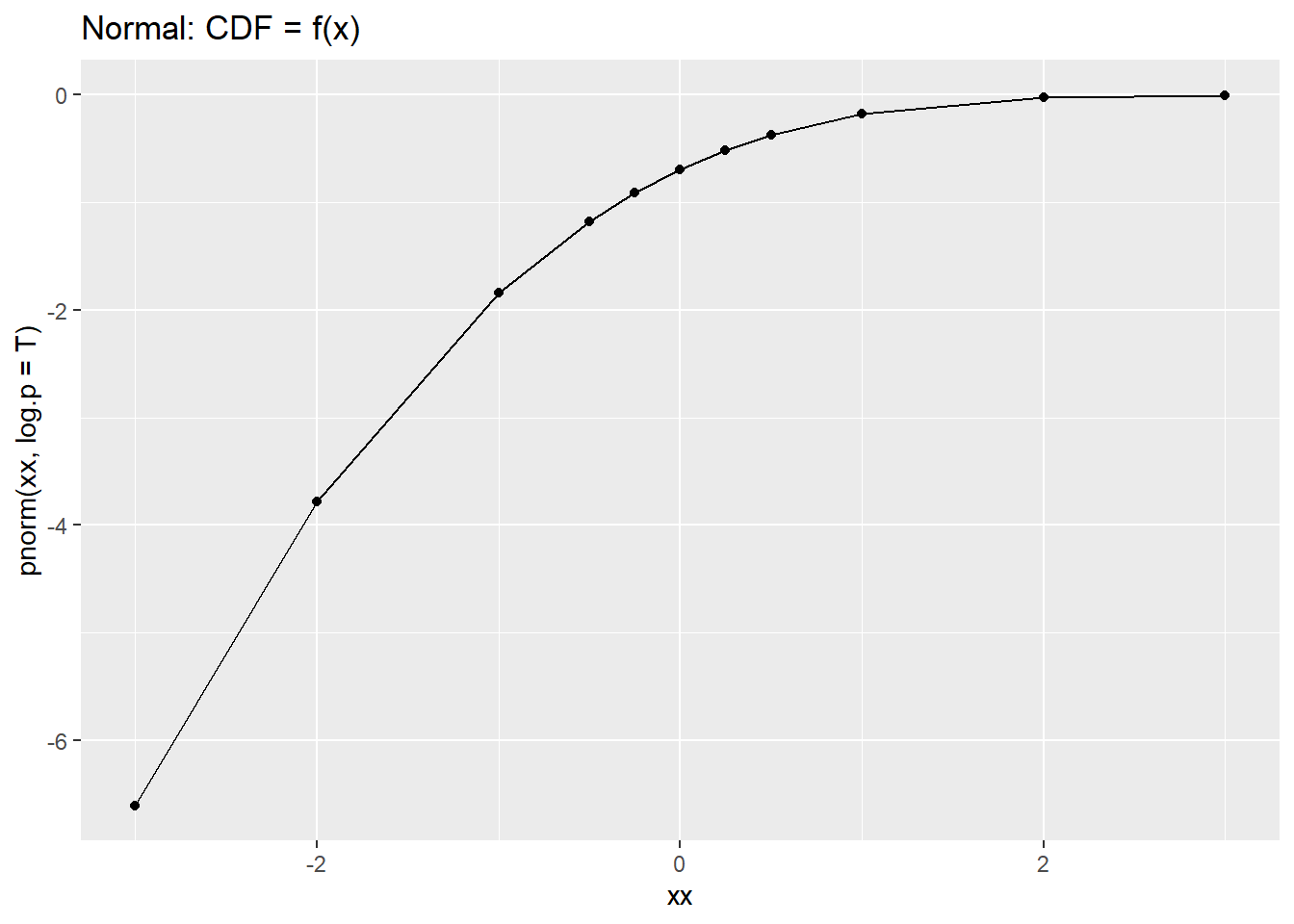qplot(xx, dlnorm(xx), geom = c("point", "line"), main = "Log Normal PDF = f(x) ")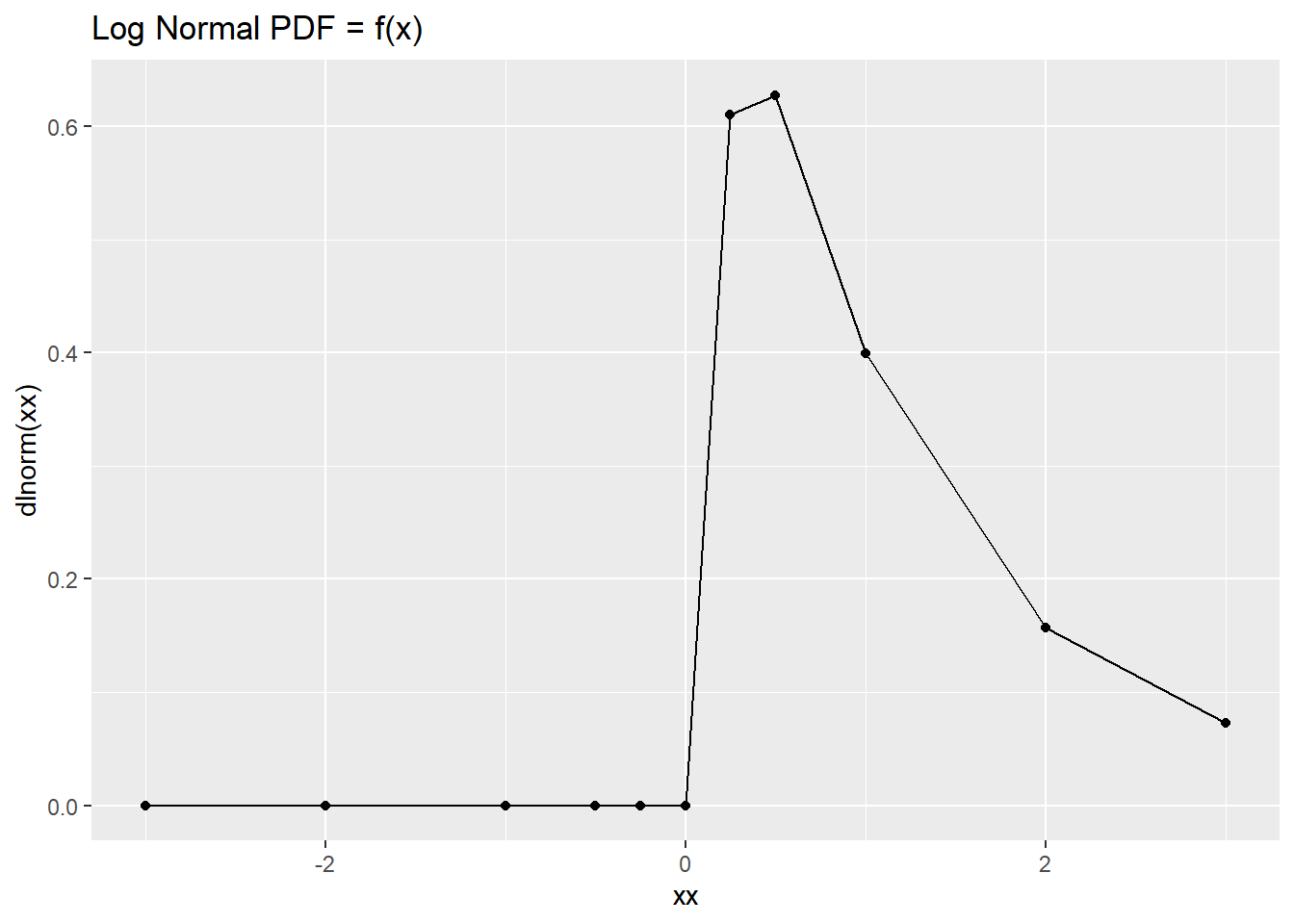qplot(xx, dlnorm(xx, log=T), geom = c("point", "line"), main = "Log Normal: PDF = f(x) ")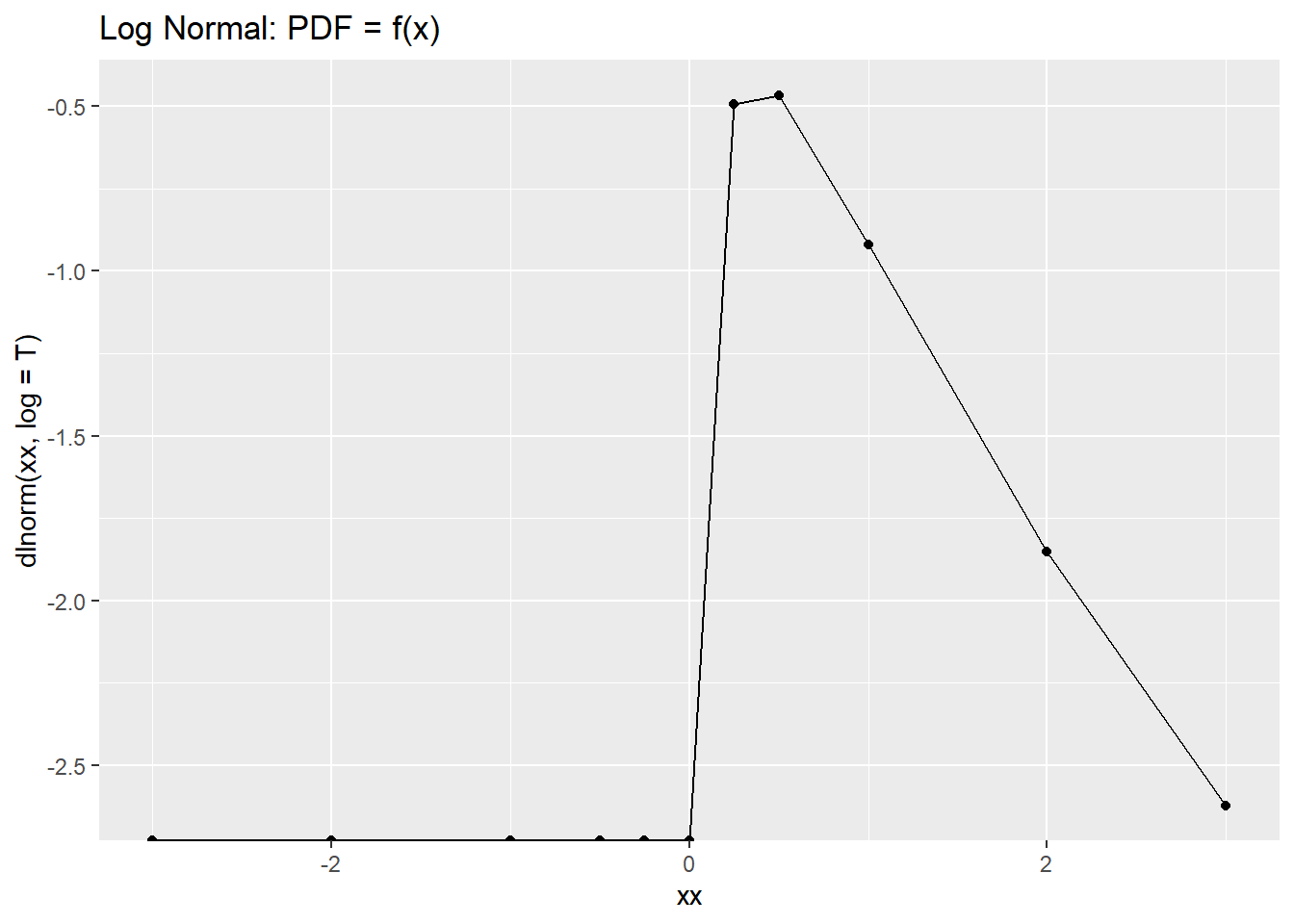qplot(xx, plnorm(xx), geom = c("point", "line"), main = "Log Normal CDF = f(x) ")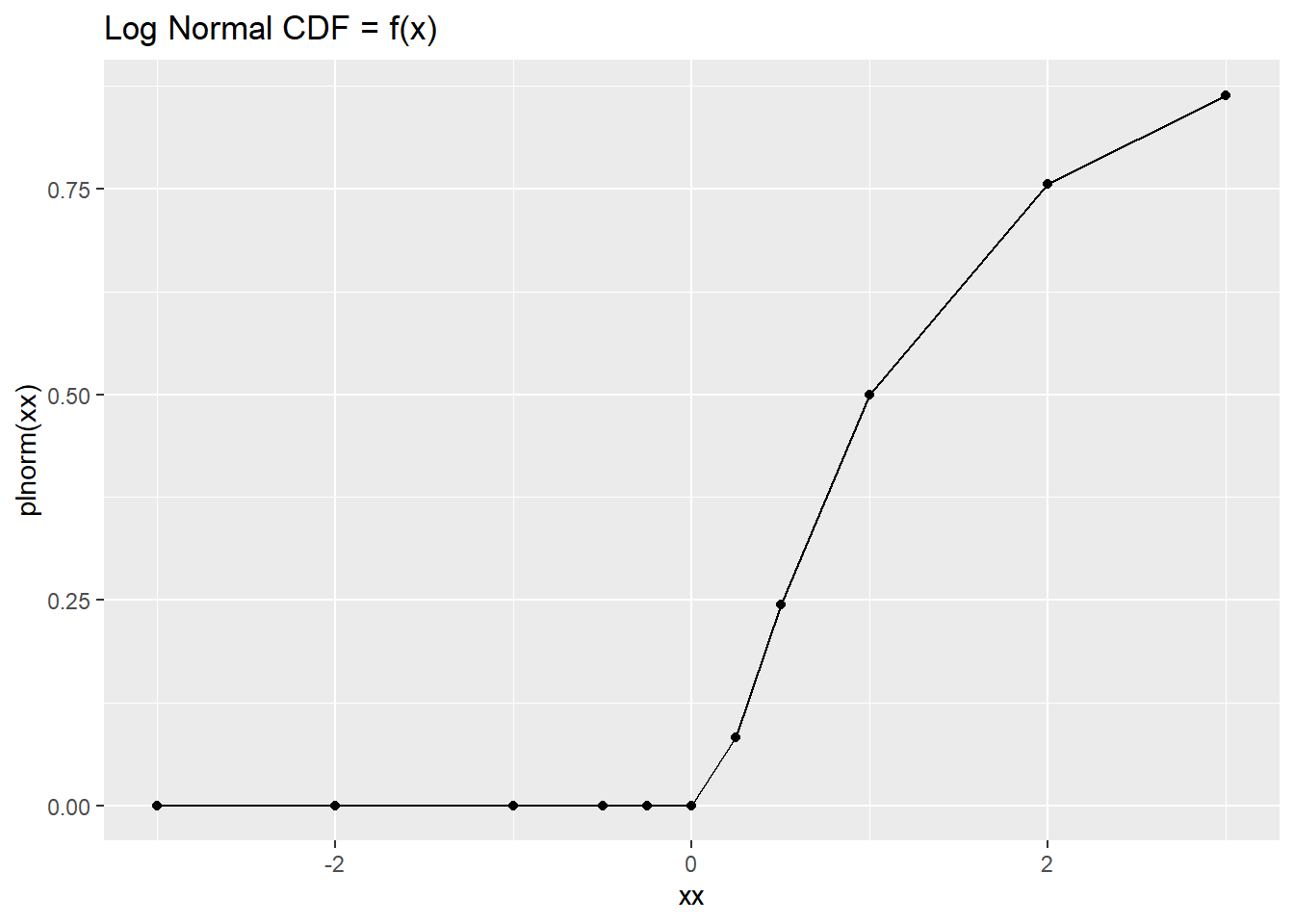qplot(xx, plnorm(xx, log.p=T), geom = c("point", "line"), main = "Log Normal: CDF = f(x) ")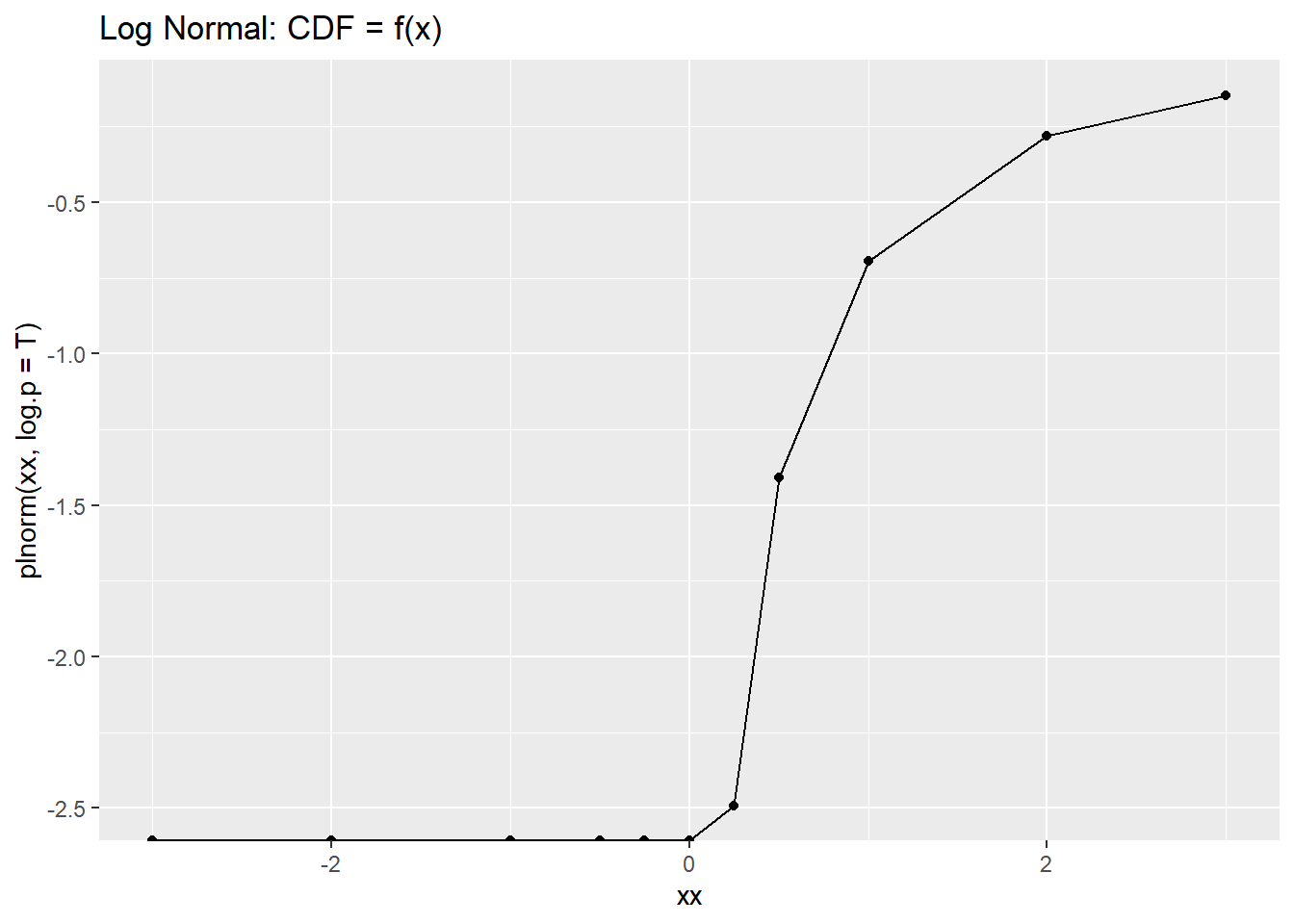Or do it in loop with all functions ! See: https://stackoverflow.com/questions/69647331/function-names-as-looping-variables-in-r

for (i in c("norm", "lnorm", "exp")) {
fun <- getFunction(paste0("d",i))
fun %>% print
qplot(xx, fun(xx), main = paste0("PDF: ", i))  %>% print
}
## function (x, mean = 0, sd = 1, log = FALSE)
## .Call(C_dnorm, x, mean, sd, log)
## <bytecode: 0x00000000137d8828>
## <environment: namespace:stats>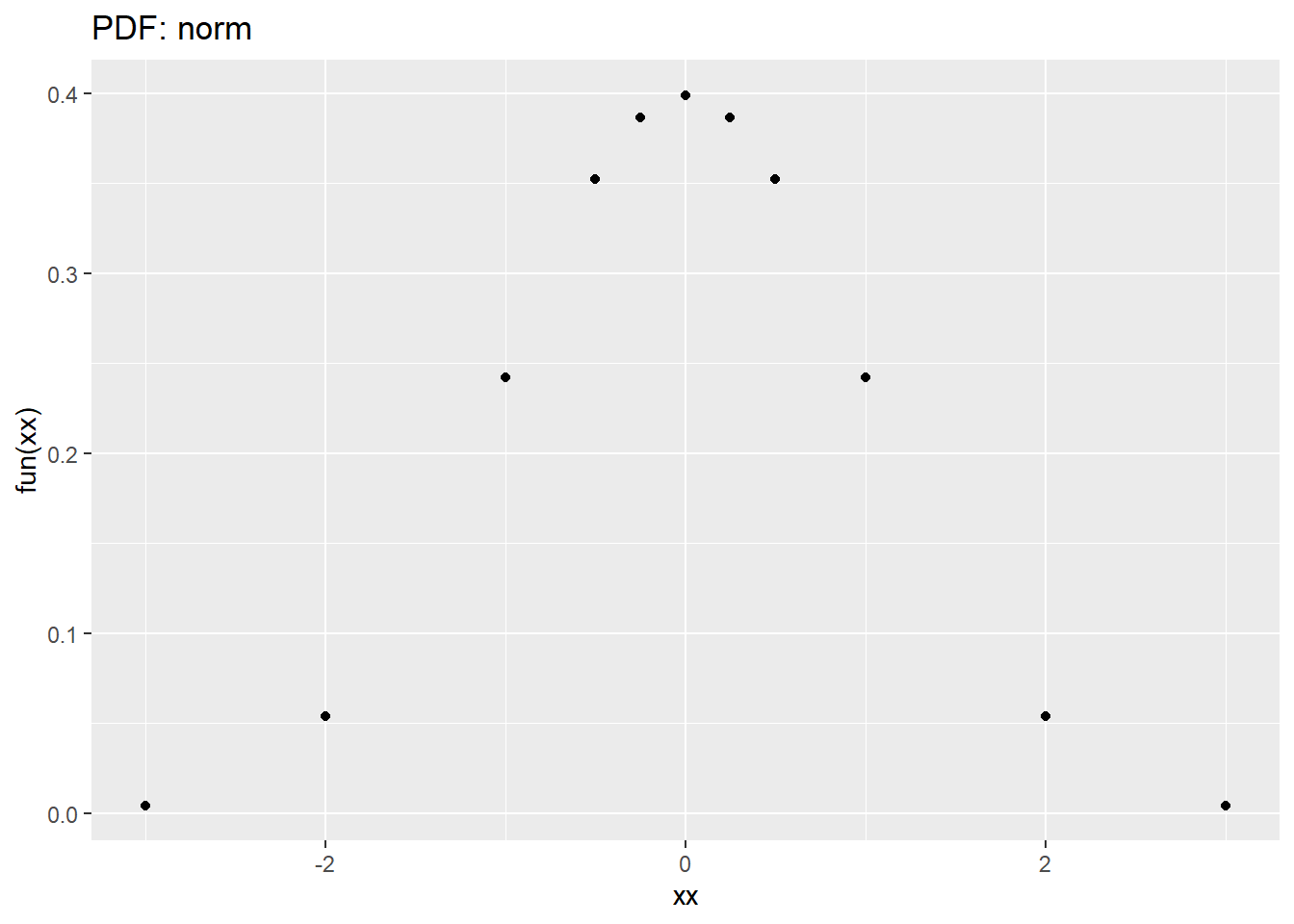## function (x, meanlog = 0, sdlog = 1, log = FALSE)
## .Call(C_dlnorm, x, meanlog, sdlog, log)
## <bytecode: 0x0000000019265598>
## <environment: namespace:stats>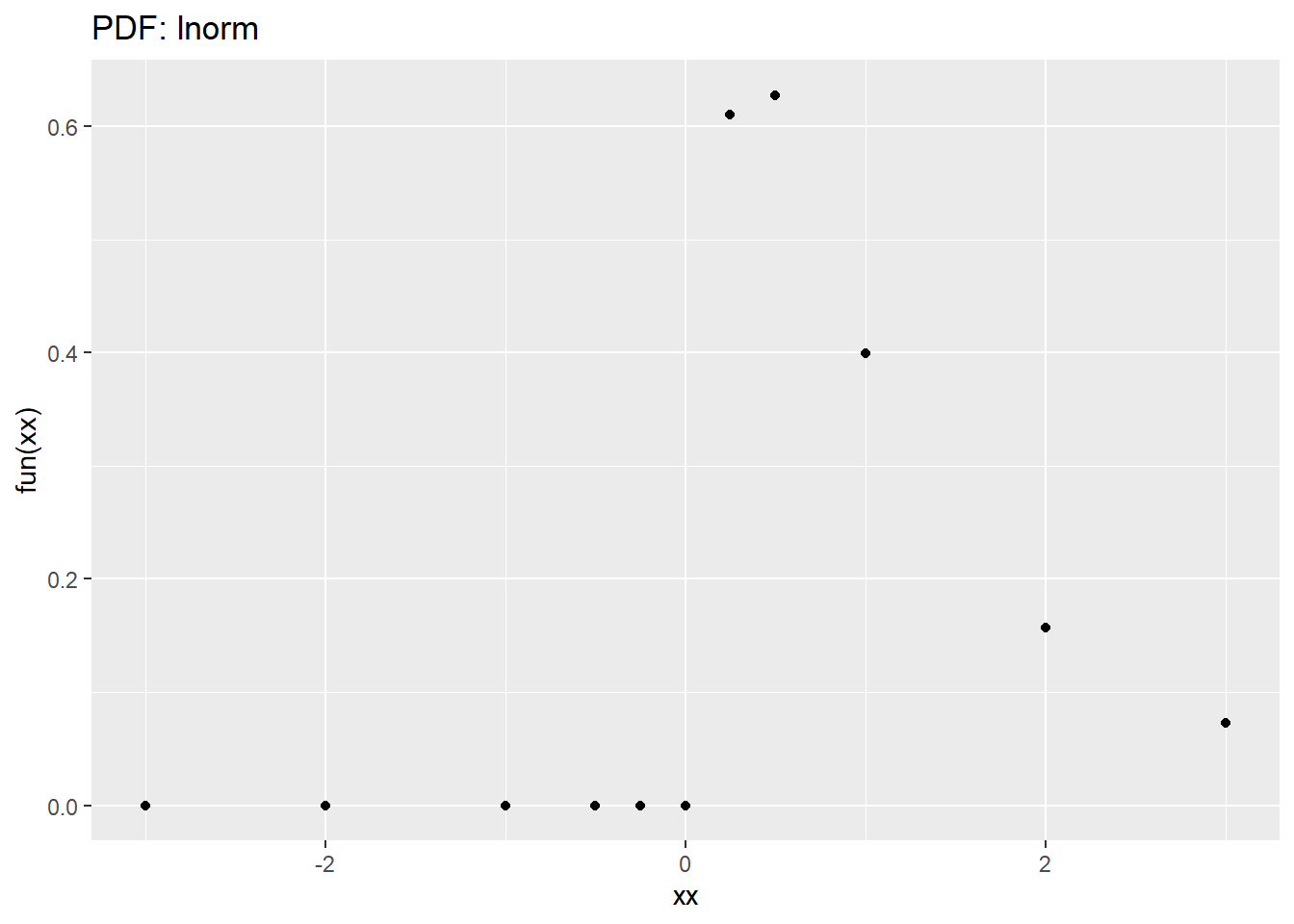## function (x, rate = 1, log = FALSE)
## .Call(C_dexp, x, 1/rate, log)
## <bytecode: 0x0000000019819658>
## <environment: namespace:stats>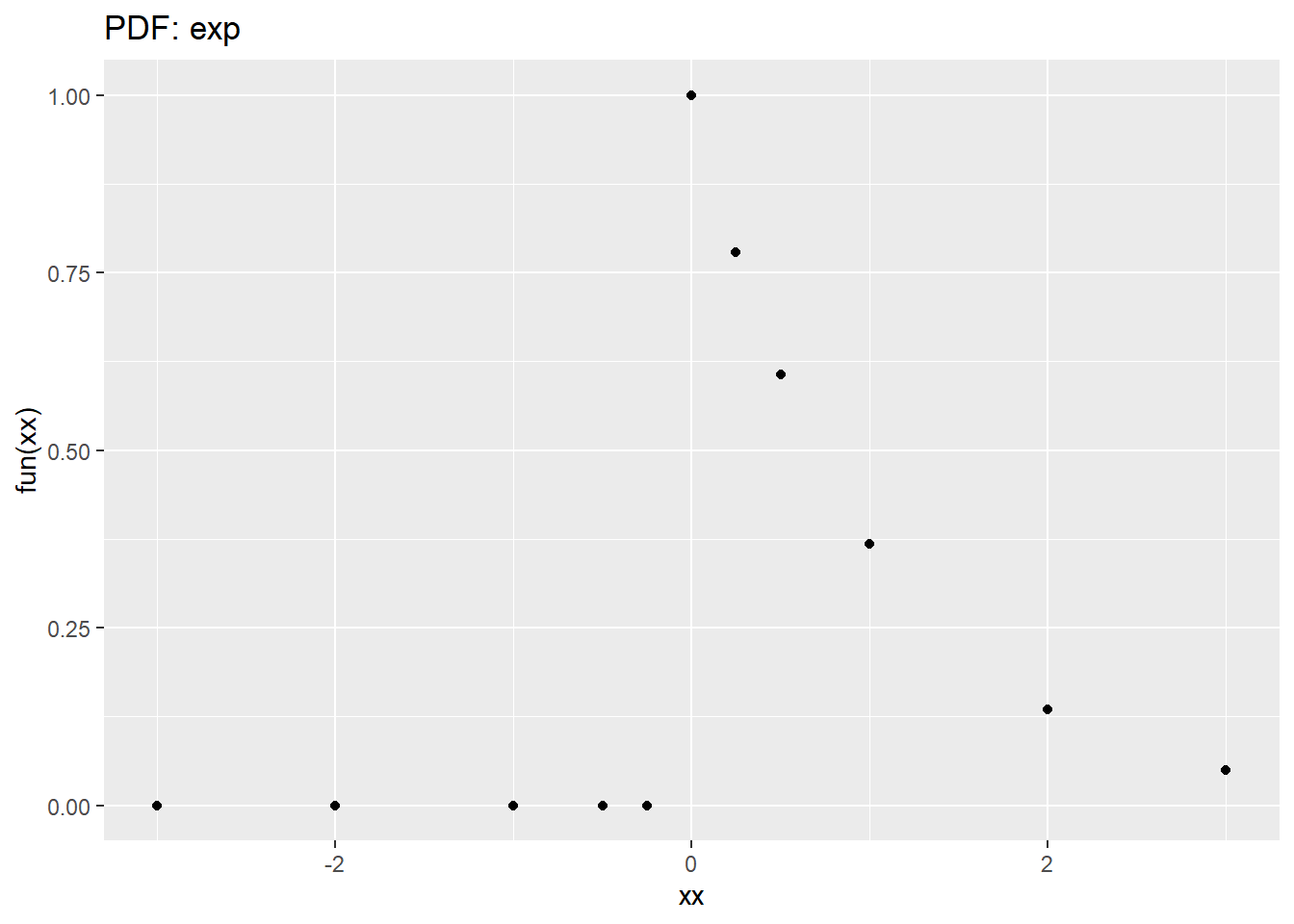## Another way to run R code inside Rmarkdown

source("stat101a.R")
##  0.5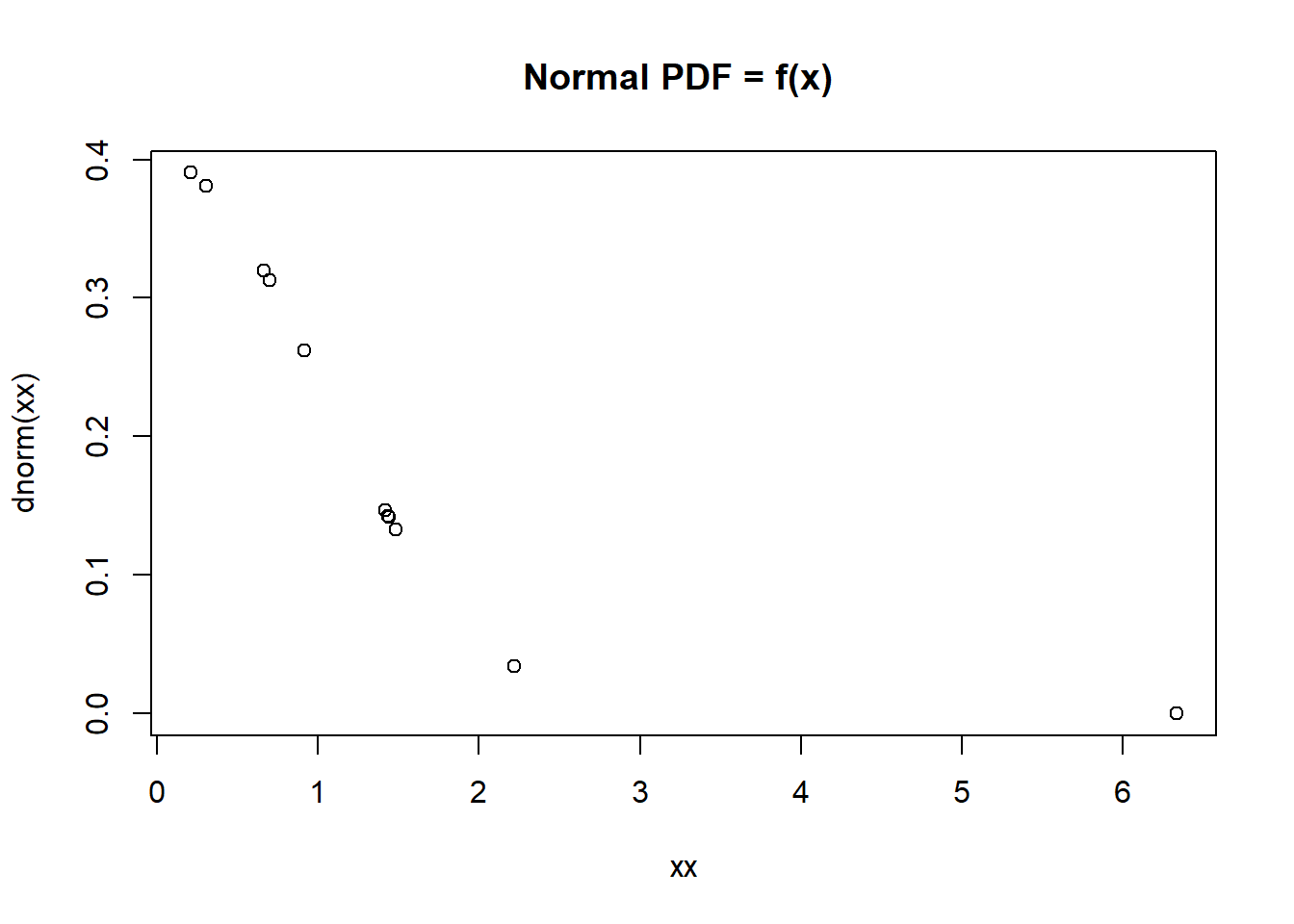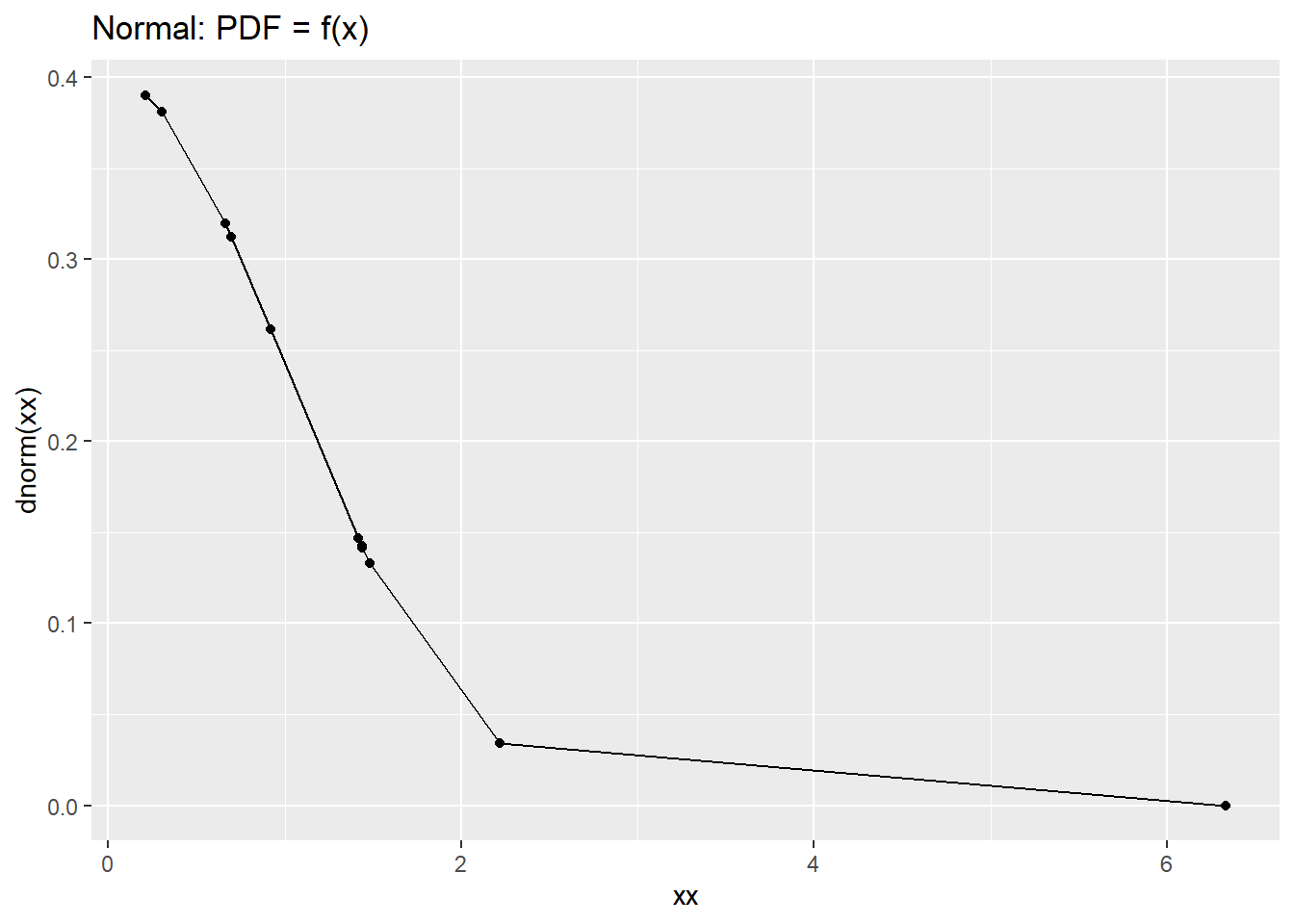## Colophon

When archiving an article that was generated from R code, it's a good practice to include the information about the system where the code was run:

sessionInfo()
## R version 4.0.2 (2020-06-22)
## Platform: x86_64-w64-mingw32/x64 (64-bit)
## Running under: Windows 10 x64 (build 18363)
##
## Matrix products: default
##
## locale:
##
## attached base packages:
##  stats     graphics  grDevices utils     datasets  methods   base
##
## other attached packages:
##  ggplot2_3.3.3  magrittr_2.0.1
##
## loaded via a namespace (and not attached):
##   bslib_0.2.4       compiler_4.0.2    pillar_1.6.0      jquerylib_0.1.3
##   tools_4.0.2       digest_0.6.25     jsonlite_1.7.2    evaluate_0.14
##   lifecycle_1.0.0   tibble_3.0.3      gtable_0.3.0      pkgconfig_2.0.3
##  rlang_0.4.10      DBI_1.1.1         yaml_2.2.1        xfun_0.22
##  withr_2.4.1       stringr_1.4.0     dplyr_1.0.5       knitr_1.29
##  generics_0.0.2    sass_0.3.1        vctrs_0.3.7       grid_4.0.2
##  tidyselect_1.1.0  glue_1.4.2        R6_2.4.1          fansi_0.4.1
##  rmarkdown_2.7     farver_2.0.3      purrr_0.3.4       scales_1.1.1
##  ellipsis_0.3.1    htmltools_0.5.1.1 assertthat_0.2.1  colorspace_1.4-1
##  labeling_0.3      utf8_1.1.4        stringi_1.4.6     munsell_0.5.0
##  crayon_1.4.1